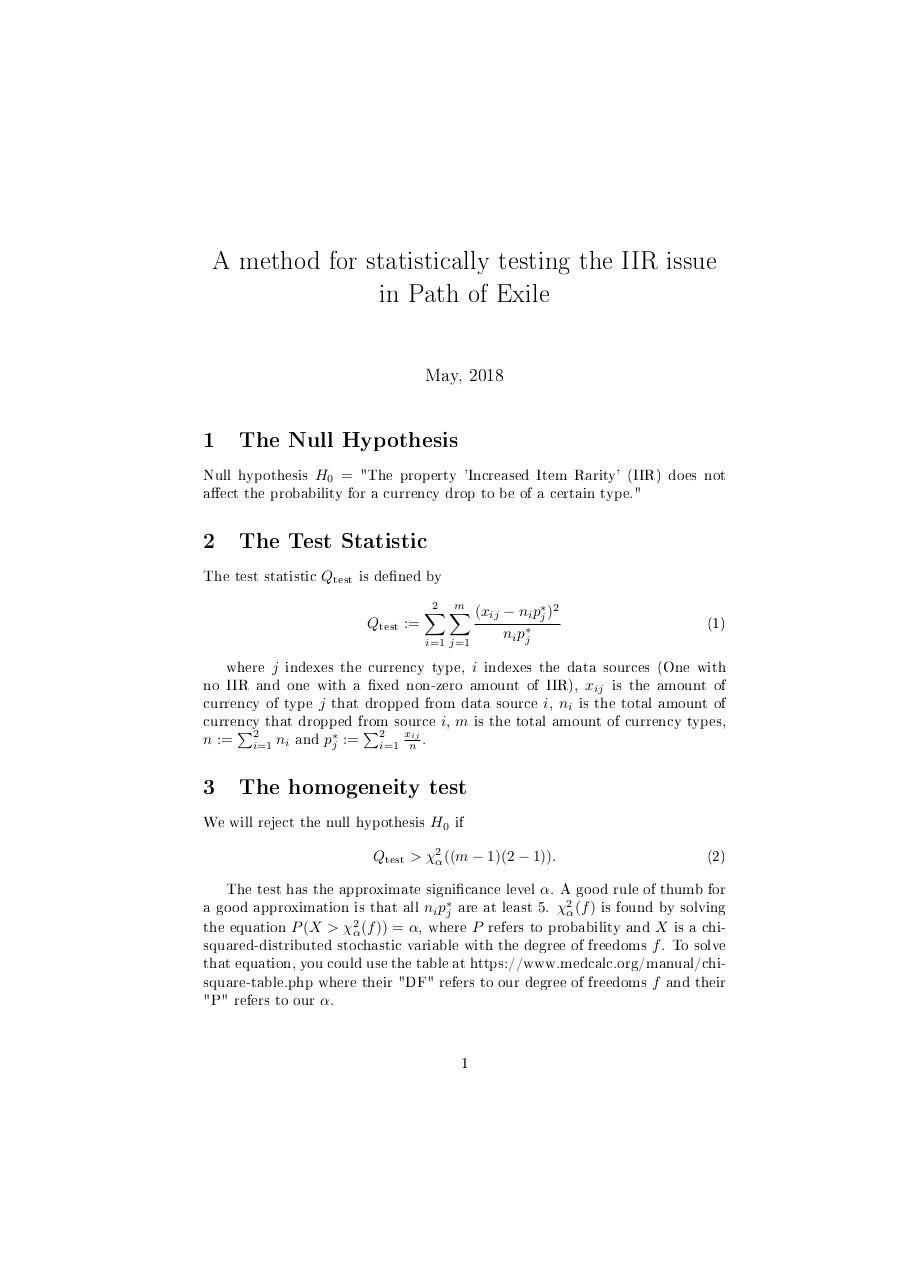gg .pdf

File information

Original filename: gg.pdf

This PDF 1.5 document has been generated by TeX / MiKTeX pdfTeX-1.40.19, and has been sent on pdf-archive.com on 04/05/2018 at 03:18, from IP address 109.225.x.x. The current document download page has been viewed 225 times.
File size: 110 KB (2 pages).
Privacy: public file

gg.pdf (PDF, 110 KB)

Document preview

A method for statistically testing the IIR issue
in Path of Exile
May, 2018

1

The Null Hypothesis

Null hypothesis H0 = "The property 'Increased Item Rarity' (IIR) does not
aect the probability for a currency drop to be of a certain type."

2

The Test Statistic

The test statistic Qtest is dened by
Qtest :=

m
2 X
X
(xij − ni p∗j )2
ni p∗j
i=1 j=1

(1)

where j indexes the currency type, i indexes the data sources (One with
no IIR and one with a xed non-zero amount of IIR), xij is the amount of
currency of type j that dropped from data source i, ni is the total amount of
currency
i, m is the total amount of currency types,
P2 that dropped from
P2 source
x
n := i=1 ni and p∗j := i=1 nij .

3

The homogeneity test

We will reject the null hypothesis H0 if
Qtest &gt; χ2α ((m − 1)(2 − 1)).

(2)

The test has the approximate signicance level α. A good rule of thumb for
a good approximation is that all ni p∗j are at least 5. χ2α (f ) is found by solving
the equation P (X &gt; χ2α (f )) = α, where P refers to probability and X is a chisquared-distributed stochastic variable with the degree of freedoms f . To solve
that equation, you could use the table at https://www.medcalc.org/manual/chisquare-table.php where their "DF" refers to our degree of freedoms f and their
"P" refers to our α.
1

4

Source of claims

The mathematical claims come from an old book in statistics that unfortunately
is not written in English. I wouldn't be surprised if there are sources in English
describing something analagous or identical.

2HTML Code

Copy the following HTML code to share your document on a Website or Blog

QR CodeRelated keywords